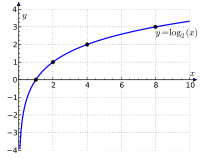# O-notation

• used to classify (compare) algorithms based on how they respond to changes in input size, i.e. how they scale
• you can’t really tell how fast an algorithm will be without benchmarking (CPU, disk, RAM complexities) but O() notation can serve as a general guide
• O is used because the growth rate of an algorithm’s run time is known as its order
• order of magnitude is related but different - how many digits are in a number describing size (1 000 is an order of magnitude larger than 100)

NOTE: Logarithms (log) mentioned here are base 2.

For example, simple search is O(n) algorithm and binary search is O(log n) algorithm. It means that if you have for example 100 (sorted) items you need to search through you need at most (worst-case scenario) 100 steps using simple search and 7 steps (guesses, operations) using binary search. Also notice that the growth rate of the binary search algorithm is much smaller.

n Simple search O(n) Binary search O(log n)
10 10 steps 4 steps
100 100 steps 7 steps
1 000 1 000 steps 10 steps

All this means that binary search is faster than simple search and it gets a lot faster as the input size increases.

# Scaling

The following terms describe how a system performs as data size grows: the system is unaffected, gets bit slower, gets slower, or gets much slower.

O(1) - constant scaling

• no matter the size of the input, performance of the system does not change
• such algorithms are rare
• ex. hash-table lookup in RAM

O(log n) - logarithmic scaling

• as the input size grows runtime grows but slowly
• ex. binary searchO(n) - linear scaling

• twice as much data requires twice as much processing time
• ex. simple search

O(n^m) - exponential scaling

• as input size grows the system slows down disproportionately
• O(n^2) - quadratic scaling: everybody says exponential when they really mean quadratic :-)
• ex. selection sort

# Resources

• TPoCSA, Appendix C
• Grokking Algorithms
• The Practice of Programming, ch2.5